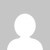# Remove strings from an Array in JavaScript | Example code

You can use the typeof() and filter() method to Remove strings from an Array in JavaScript.

## Example Remove Strings, Keep Numbers In Array With JavaScript

HTML example code – Using ES6 Arrow Function

``````<!DOCTYPE html>
<html>
<body>

<script>
function filter_list(l) {
return l.filter(x => typeof x === "number");
}
console.log(filter_list([1,2,'a','b']))

</script>

</body>
</html>``````

Output:

Without Arrow Function

``````function filter_list(l) {
return l.filter(function(x){
return typeof x === "number"
});
}
console.log(filter_list([1,2,'a','b']))``````

Using Simple Loops (for-loop)

``````function filter_list(l) {
let newArr = [];
for(let i = 0;i<l.length;i++){
if(typeof l[i] === "number") newArr.push(l[i]);
}
return newArr
}
console.log(filter_list([1,2,'a','b']))``````

Source: stackoverflow.com/

Do comment if you have any doubts or suggestions on this JS Array code.

Note: The All JS Examples codes are tested on the Firefox browser and the Chrome browser.

OS: Windows 10

Code: HTML 5 Version

## 1 thought on “Remove strings from an Array in JavaScript | Example code”

1.Write a function that takes an array of strings and removes the strings that
contain a number in returns the new array ( not the reference ).

Example: removeNumberStrings ( [“ This”,”is” , “a3” , “samp7e”, “stri9ng”]) returns [“This”, “is”]
How to solve this using arrow functions…

This site uses Akismet to reduce spam. Learn how your comment data is processed.